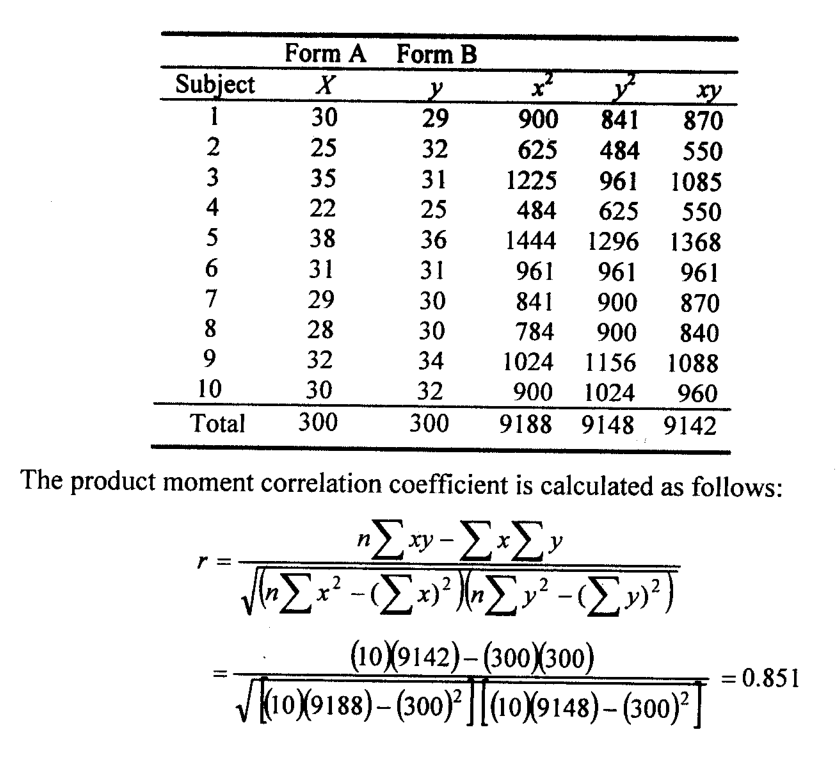# Parallel Forms Method: Definition, Example

In the parallel-forms method, two tests that are equivalent in the sense that they contain the same kinds of items of equal difficulty but not the same items are administered to the same individuals. This technique is also referred to as the method of equivalent forms.

We illustrate the computation and interpretation of parallel form reliability by another example due to Sprinthall.

As the name applies, the technique uses two parallel forms, A and B, and these are administered to the same group, one test immediately or fairly soon following the other.

The two test-forms of the same test are said to be parallel or equivalent because they are made up of the same types of items covering the same materials or the same types of information.

These forms are so made that if one form correlates to a certain extent with some other measures, then the other form also correlates to the same degree.

Pearson’s product-moment correlation coefficient (r) is then computed to find the degree of equivalence between these two forms. The computed coefficient is called the coefficient of equivalence.

Suppose, for example, the scores of 100 persons on two equivalent forms “A” and “B” of a ‘word fluency test’ were correlated.

In form “A,” the persons were given five minutes to write as many words as they could that began with a given letter, say, ‘s’.

The form “B”t was identical to “A,” except that a different letter, say, ‘r’ was employed. The two letters were chosen by the experimenter as being approximately equal in difficulty for this purpose. A correlation coefficient can then be computed, and its statistical significance is tested.

## Example of Parallel Forms Method

The Sound Recognition Test is a test for a condition known as auditory agnosia, or a person’s ability to recognize familiar environmental sounds, such as a bell, a whistle, or crowd’s sounds.

There are two forms of the test, A and B, with a total of 13 items per test. Scoring is based on allowing up to 3 points per item, making 39, the highest possible score.

A group of normal, five-year-old children was selected and given form A. Then, the next day, they were given form B. The accompanying table shows the data and the scheme of calculating the reliability coefficient.With 8 df, the product-moment correlation coefficient r is significant at 5% level (a table value of .632 is required). Thus the parallel form reliability is established

Under the test-retest method, data were collected under system-1 by the registrar on a regular basis.

This system was referred to as ‘test.’ Under system-2, retrospective data for the last three months were collected by the supervisors, which they called retest (BBS, 2004: ill).

We now turn to discuss internal consistency techniques.

These techniques are referred to as internal consistency technique because what is being assessed in this case is the consistency that occurs within the test rather than between two administrations of the same test or separate administration of the alternate forms.

The methods to be discussed are

Related Posts ⁄